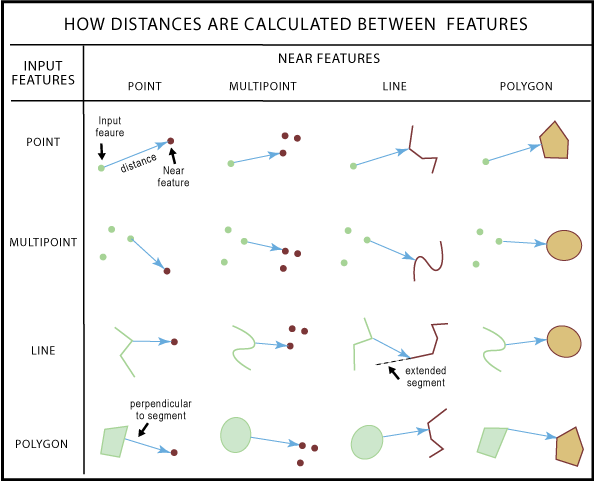# How proximity tools calculate distance

## How distance is defined

The distance between any two features is calculated as the shortest separation between them, that is, where the two features are closest to each other. This logic is applied by any geoprocessing tool that calculates distance, including tools such as Near, Generate Near Table, and Spatial Join (with CLOSEST match option).

Distance measurements will be most accurate when your input data is in an equidistance projected coordinate system. While distance calculations can always be performed regardless of coordinate system, the results may be inaccurate or even meaningless when your data is in a geographic coordinate system or an improperly selected projected coordinate system.

For the discussion below, distance will always refer to the smallest separation between two features.

## Special considerations

• Multiple features may be equally closest to another feature. When this occurs, one of the equally closest features is randomly selected as the closest.
• When one feature contains or is within another feature, the distance between them is zero.
• This means that when a feature is inside a polygon, the distance between the feature and the surrounding polygon is zero.
• The distance between two features is zero whenever there is at least one x,y coordinate that is shared between them.
• This means that when two features intersect, overlap, cross, or touch, the distance between them is zero.
• Distance is always calculated to the boundary of a polygon feature, not to the center or centroid of the polygon.
• As noted above, if a feature is completely inside a polygon, the distance between the feature and the surrounding polygon is zero.
• The distance between two features (of any type) is always the same irrespective of which one is being measured to and from.

## Basic operations for finding distance

Calculating distance is dependent on the geometry type of the features as well as other factors such as coordinate system. However, there are three basic rules, described in detail below, that determine how distance is calculated.

1. The distance between two points is the straight line connecting the points.
2. Distance from a point to a line is either the perpendicular or the closest vertex.
3. Distance between polylines is determined by segment vertices.

## Rule 1: The distance between two points is the straight line connecting the points

The following illustration shows the distance between two points along with several other keywords and features used by the proximity tools.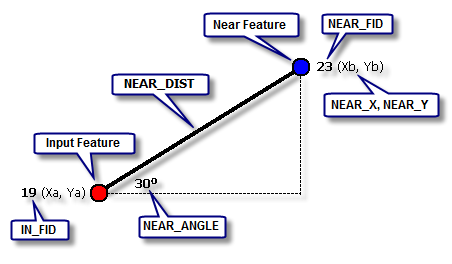The keywords in the callouts above (IN_FID, NEAR_DIST, NEAR_FID, NEAR_X, NEAR_Y, and NEAR_ANGLE) are fields added to the output by the Generate Near Table and tools, and to the input feature class when the Near tool is run.

### Multipoint to multipoint

For the special case of calculating distances between multipoints, distances from each point of an input multipoint feature to each point of the near multipoint is calculated using Rule 1, and the smallest of these distances is the distance between the two multipoint features.

Additionally, when one of the multipoint's points is on top of one of the points for another multipoint, the distance between the two multipoint features is zero. This applies to all multipart features.

## Rule 2: Distance from a point to a polyline is the perpendicular or the closest vertex

In ArcGIS, line features are referred to as polylines. These two terms, line and polyline, are interchangeable. A polyline is an ordered collection of points, and these points are referred to as vertices. An individual vertice is a vertex. A polyline can have any number of vertices. The line defined by two vertices is called a line segment, or a segment. The two vertices that define a line segment are referred to as end vertices.

Similarly, a polygon is an enclosed area defined by one or more polylines.

The shortest distance from a point to a line segment is the perpendicular to the line segment. If a perpendicular cannot be drawn within the end vertices of the line segment, then the distance to the closest end vertex is the shortest distance.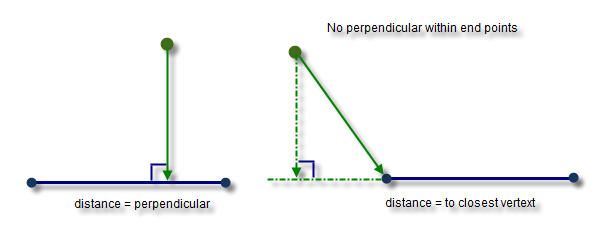### Point to Polyline

If the polyline has only one line segment, Rule 2 is applied to get the distance.

When the polyline has multiple line segments (the most common case), the closest line segment to the point is determined first, and then Rule 2 is applied to get the distance.

### Point to Polygon

Because a polygon is an area enclosed by an ordered collection of line segments, calculating the distance from a point to a polygon involves identifying the closest line segment to the point, and then Rule 2 is applied to get the distance.

The distance is positive only when the point is outside of the polygon; otherwise, it is zero.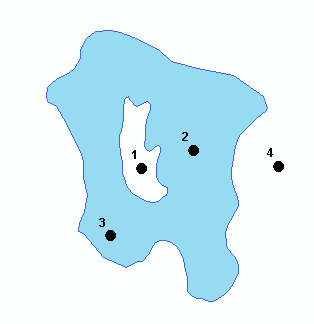In the above illustration, distance is zero for points 2 and 3 and positive for points 1 and 4.

## Rule 3: Distance between polylines is determined by line segment vertices

For two nonpoint features such as two line segments:

1. The distance from each of the end vertices of the input segment to the near segment is calculated using Rule 2.
2. The distance from each of the end vertices of the near segment to the input segment is calculated.
The smaller of the these two distance values is the distance between two segments.

### Polyline to Polyline

In the simplest case, assume both polyline features have one segment each. The illustration below shows the perpendicular, CX, from vertex C to the segment defined by vertices AB. A perpendicular from vertex D can also be computed, but its distance is greater than CX. Thus, CX is the shortest distance from segment CD to segment AB.

Note that no perpendicular can be drawn from vertex A or B to segment CD, so the shortest distance is computed from vertices A and B to vertex C. The result is that AC is the shortest distance from segment AB to segment CD.

Of the two distances computed (AC and CX), CX is the shortest distance between two segments as it is the smallest of all vertex-to-segment distances.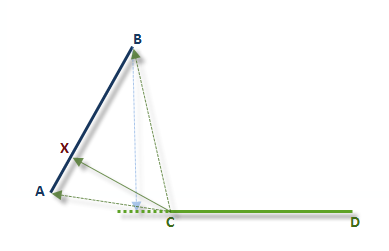When both polylines have multiple segments, the two closest segments are found then the distance between them is calculated as per Rule 3.

### Polyline to Polygon

When computing the distance between a polyline and a polygon, the two closest segments are identified: one from the polyline and the other from the sequence of segments composing a polygon boundary. The distance between these two segments is calculated following the process described in Rule 3.

## Summary

The following diagram gives an overall picture of how distances are calculated between different feature types and where the closest locations can be as described above. Not all possible combinations are shown.